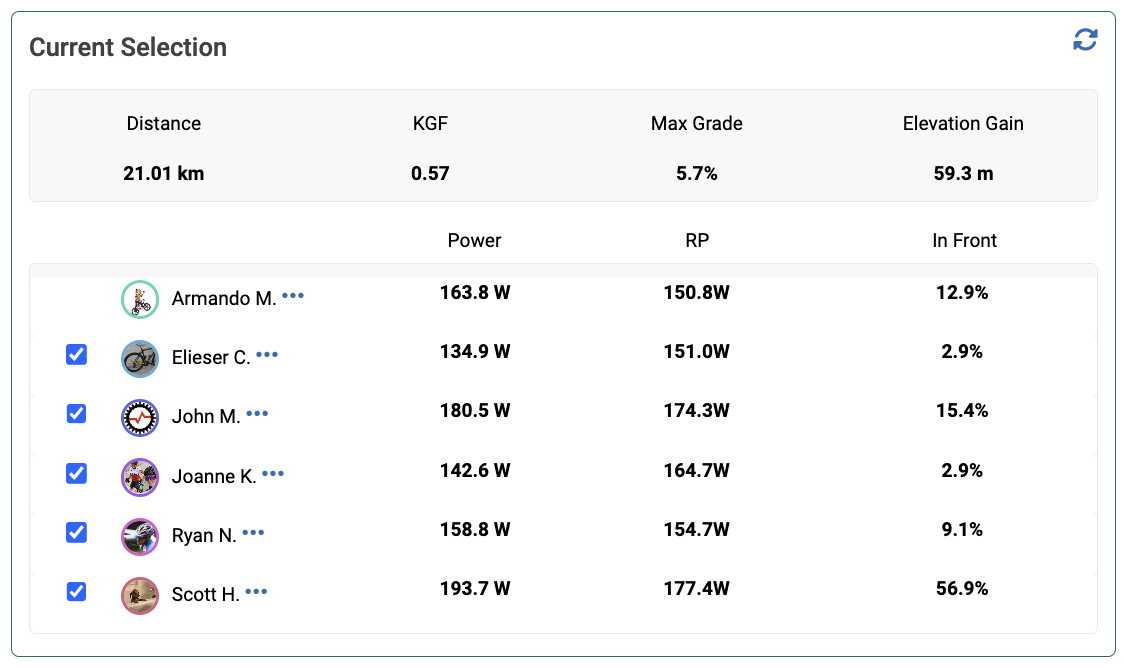Relative Power (RP) quantifies how much power a standard size (75kg) cyclist would need to produce in order to match the power of another rider, all else being equal*.  The calculation used in Xert is:

`Relative Power = Power * (75/mass)^k`

where mass is the weight in kilograms of the other rider and k is the Kilogram Factor (KGF).  KGF is determined based on the gradient.  The higher the gradient, the higher the KGF value.

For example, if a 65kg rider is generating 300W where the KGF is 0.7, the Relative Power is 300*(75/65)^0.7 = 332W.

* A rider can improve their Relative Power by reducing weight but also by improving other factors such as aerodynamics, rolling resistance, bike weight, and position on the bike.